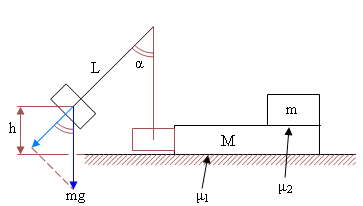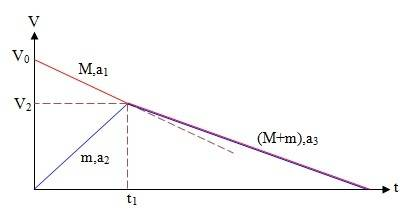# A mass hits other two, one on top of the other

## Homework Statement[/B]
Mass m is raised to height h and released. it hits, elastically, mass M which is on a surface with friction coefficient μ1. mass m, same as the first, lies on top of M. the coefficient between them is μ2.
1) What's the box's velocity just before the hit
2) What is the tension T1 in the rope immediately after releasing
3) What is the tension T2 in the rope just before the hit
4) What is M's acceleration immediately after the hit
5) What is m's acceleration (the box on top) immediately after the hit
6) How much time M travels till it stops
7) Draw on one coordinate system M and m's velocities till they halt, relative to the floor
8) What distance M travels till it halts
9) What should be the minimal length of M so that m won't fall

## Homework Equations

Potential energy of height: ##EP=mgh##
Kinetic energy: ##EK=\frac{1}{2}mv^2##
Conservation of momentum: ##m_1v_1+m_2v_2=m_1v_1'+m_2v_2'##
Acceleration, distance and speed in constant acceleration: ##V^2=V_0^2+2ax##
Centripetal force: ##F_c=\frac{mv^2}{r}##

## The Attempt at a Solution

Height transformed into speed:
$$mgh=\frac{1}{2}mv^2~~\rightarrow~~v_2=2gh$$
Tension before release:
$$T_1=mg\cos\alpha=mg\frac{l-h}{l}$$
Tension before hit:
$$F_c=\frac{mv^2}{r}~~\rightarrow~~T_2=m\left[ \frac{v^2}{l}+g \right]=mg\left( \frac{2h}{l}+1 \right)$$
Conservation of momentum and energy, V is M's velocity:
$$\left\{ \begin{array}{l} \frac{1}{2}mv^2=\frac{1}{2}\left[ mv_1^2+MV_0^2 \right] \\ mv=MV_0-mv_1 \end{array}\right.$$
$$\rightarrow V_0=\frac{m\sqrt{2gh}\left[ M+\sqrt{M(2M+m)} \right]}{M(M+m)}$$
M's acceleration after the hit:
$$(M+m)g\mu_1+mg\mu_2=Ma_1~~\rightarrow~~a_1=\frac{g\left[ (M+m)\mu_1+m\mu_2 \right]}{M}$$
m's acceleration after the hit:
$$mg\mu_2=ma_2~~\rightarrow~~a_2=g\mu_2$$
The time till equality of velocities is t1:
$$V_0-a_1t_1=a_2t_1:~~V_0-\frac{(M+m)\mu_1+m\mu_2}{M}gt_1=g\mu_2t_1$$
$$\rightarrow t_1=\frac{MV_0}{(M+m)(\mu_1+\mu_2)g}$$
Their common velocity, after time t1:
$$V_2=a_2t_1=g\mu_2\frac{MV_0}{(M+m)(\mu_1+\mu_2)g}=\frac{MV_0\mu_2}{(M+m)(\mu_1+\mu_2)}$$
When they move at one velocity, their common deceleration a3 is (f=friction force):
$$f=(M+m)a_3~~\rightarrow~~(M+m)g\mu_1=(M+m)a_3~~\rightarrow~~a_3=g\mu_1$$
Time till both stop t2:
$$0=V_2-a_3t_2: ~~\frac{MV_0\mu_2}{(M+m)(\mu_1+\mu_2)}=g\mu_1t_2$$
$$\rightarrow t_2=\frac{MV_0\mu_2}{(M+m)(\mu_1+\mu_2)\mu_1g}$$
Total time to full stop:
$$t_{tot}=t_1+t_2=\frac{MV_0}{(M+m)(\mu_1+\mu_2)g}\left( 1+\frac{\mu_2}{\mu_1}\right)$$
The graph:to check which line is more inclined, i compare a1 to a3:
$$a_1=\frac{g\left[ (M+m)\mu_1+m\mu_2 \right]}{M}=\left[ \frac{M+m}{M}\mu_1+\frac{m}{M}\mu_2 \right]g>a_3=\mu_1g$$
The distance M travels till equality of velocities: ##V_2^2=V_0^2-2a_1x_1##
$$\frac{\mu_2^2M^2V_0^2}{(M+m)^2(\mu_1+\mu_2)^2}=V_0^2-2\frac{g\left[ (M+m)\mu_1+m\mu_2 \right]}{M}X_1~~\rightarrow~~x_1=...$$
The distance M travels from the equality till it stops: ##0=V_2^2-2A_3x_2##
$$0=V_0^2-2g\mu_1x_3~~\rightarrow~~x_2=...$$
$$x_{tot}=x_1+x_2$$
But the expressions are complicated.
The time till M stops:
M's absolute velocity+m's relative velocity yields m's absolute velocity: $$V_{M(abs)}+v_{m(rel)}=v_{m(abs)}$$
$$\rightarrow~v_{m(rel)}=v_{m(abs)}-V_{M(abs)}=a_2t-(V_0-a_1t)=g\mu_2t-\left( V_0-\frac{(M+m)\mu_1+m\mu_2}{M}gt \right)$$
By equating ##v_{m(rel)}## to 0 i find t, the time till m stops (equal velocities):
$$v_{m(rel)}=0~~\rightarrow~~t=\frac{MV_0}{g[(M+m)(\mu_1+\mu_2)]}$$
The distance traveled by m: ##x_1=\frac{1}{2}a_{m(rel)}t^2##:
$$a_{m(rel)}=a_{m(abs)}-V_0=g\mu_2-\frac{(M+m)\mu_1+m\mu_2}{M}g=\frac{(M-m)\mu_2-(M+m)\mu_1}{M}g$$
The distance x1 involves the square of the above t, and it's only a part of the total distance M travels. the second part, x2, from ##0=V_2^2-2a_3x_2##
It's tiring, maybe there's a simpler way, for some of the stages?

It would appear from the diagram that you would need to express the height of the mass on the string in terms of alpha.

can someone else have a look?

haruspex
Homework Helper
Gold Member
Your expression for V0 is wrong. It should be much simpler.

•Karol
Momentum ##P=mv=m\cdot EK=m\cdot EP## in our case:
$$\left\{ \begin{array}{l} mgh=\frac{1}{2}\left[ mv_1^2+MV_0^2 \right] \\ m\cdot mgh=MV_0-mv_1 \end{array}\right.$$
But that still gives a complicated V0.

I don't see which other principles i can use except for conservation of momentum and energy in order to find V0

The COM velocity:
$$V_c=\frac{m_1v_1+m_2v_2}{m_1+m_2}$$
In our case:
$$V_0=2V_c=\frac{2m\sqrt{2gh}}{M+m}$$
Is the rest O.K.?
Their common velocity, after time t1:
$$V_2=\frac{MV_0\mu_2}{(M+m)(\mu_1+\mu_2)}=\frac{2Mm\sqrt{2gh}}{(M+m)^2(\mu_1+\mu_2)g}$$
Time till both stop t2:

Last edited:
haruspex
Homework Helper
Gold Member
The COM velocity:
$$V_c=\frac{m_1v_1+m_2v_2}{m_1+m_2}$$
In our case:
$$V_0=2V_c=\frac{2m\sqrt{2gh}}{M+m}$$
Is the rest O.K.?
Yes, that all looks good except I did not check the part after "but the expressions are still complicated". I assume from there you are answering (9), since you already have the answers for all the other parts. For part (9), you know the initial relative velocity, V0, the final relative velocity, 0, and the relative acceleration, a1+a2. You can get the relative distance straight from those.

Total time to full stop:
$$t_{tot}=t_1+t_2=\frac{MV_0}{(M+m)(\mu_1+\mu_2)g}\left( 1+\frac{\mu_2}{\mu_1}\right)$$
The expressions for the distances M travels till stop: x1 and x2 are very tiring.
m's relative acceleration, i found in post #1, is:
$$a_{m(rel)}=a_{m(abs)}-a_M=g\mu_2-\frac{(M+m)\mu_1+m\mu_2}{M}g=\frac{(M-m)\mu_2-(M+m)\mu_1}{M}g$$
M's minimal length x:
$$0=V_0^2-2a_{m(rel)}\cdot x~~\rightarrow~~x=\frac{V_0^2}{2a_{m(rel)}}$$
$$x=\frac{2M^2m\sqrt{2gh}}{[(M-m)\mu_2-(M+m)\mu_1](M+m)2g}$$

haruspex
Homework Helper
Gold Member
Total time to full stop:
$$t_{tot}=t_1+t_2=\frac{MV_0}{(M+m)(\mu_1+\mu_2)g}\left( 1+\frac{\mu_2}{\mu_1}\right)$$
The expressions for the distances M travels till stop: x1 and x2 are very tiring.
m's relative acceleration, i found in post #1, is:
$$a_{m(rel)}=a_{m(abs)}-a_M=g\mu_2-\frac{(M+m)\mu_1+m\mu_2}{M}g=\frac{(M-m)\mu_2-(M+m)\mu_1}{M}g$$
M's minimal length x:
$$0=V_0^2-2a_{m(rel)}\cdot x~~\rightarrow~~x=\frac{V_0^2}{2a_{m(rel)}}$$
$$x=\frac{2M^2m\sqrt{2gh}}{[(M-m)\mu_2-(M+m)\mu_1](M+m)2g}$$
I thought you calculated the two absolute accelerations with opposite directions as positive, so isn't the relative acceleration the sum, not the difference?

$$a_{m(rel)}=a_{m(abs)}-a_M=g\mu_2-\left( -\frac{(M+m)\mu_1+m\mu_2}{M}g \right)=\frac{(M+m)(\mu_1+\mu_2)}{M}g$$
$$0=V_0^2-2a_{m(rel)}\cdot x~~\rightarrow~~x=\frac{V_0^2}{2a_{m(rel)}}=\frac{4Mm^2gh}{(M+m)^3(\mu_1+\mu_2)g}$$

haruspex
Homework Helper
Gold Member
$$a_{m(rel)}=a_{m(abs)}-a_M=g\mu_2-\left( -\frac{(M+m)\mu_1+m\mu_2}{M}g \right)=\frac{(M+m)(\mu_1+\mu_2)}{M}g$$
$$0=V_0^2-2a_{m(rel)}\cdot x~~\rightarrow~~x=\frac{V_0^2}{2a_{m(rel)}}=\frac{4Mm^2gh}{(M+m)^3(\mu_1+\mu_2)g}$$
Looks right. You can cancel the 'g's.
Note that as M tends to zero, x tends to zero. That is clearly not right in practice. Do you see why the discrepancy?

Note that as M tends to zero, x tends to zero. That is clearly not right in practice. Do you see why the discrepancy?
$$a_M=\frac{(M+m)\mu_1+m\mu_2}{M}g$$
When M diminishes aM increases and makes x smaller. it happens in reality, why do you say it's not right in practice?

haruspex
Homework Helper
Gold Member
$$a_M=\frac{(M+m)\mu_1+m\mu_2}{M}g$$
When M diminishes aM increases and makes x smaller. it happens in reality, why do you say it's not right in practice?
It certainly strikes me as weird that making M massless means that the mass m resting on it would stay with it. The explanation is that in reality the swinging mass m does not cease to exist as soon as it strikes M. By pretnding that it does, M retains no momentum/energy and immediately comes to a stop because of the friction. In practice, the swinging m would keep pushing it along.

So aM becomes smaller also by the same explanation, that M retains less momentum/energy?
And:
$$a_M=\frac{(M+m)\mu_1+m\mu_2}{M}g$$
Describes what really happens until M tends to zero, only then the swinging mass would continue pushing it, right?

haruspex
$$a_M=\frac{(M+m)\mu_1+m\mu_2}{M}g$$